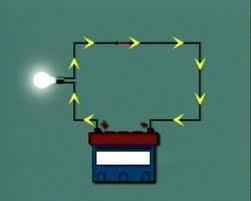•• # Electromotive Force

Electromotive force is the electrical potential energy that causes current to flow through a circuit. It results from the difference in charge between two points in a material. This difference is created when an external source such as a battery makes electrons move to both points so that there is an excess at the first point and a deficiency at the other. The volt is the unit that describes the energy that the source of electric power supplies that drives the unit charge within or around the circuit. Electromotive force was first discovered through the study of static electricity.

## How is Electromotive Force Expressed?

The equation that represents electromotive force is E = V + Ir. In this equation, V is the terminal voltage, I is the circuit amperes, and r is the internal resistance measured in ohms. By definition, a terminal’s total voltage is less than the circuit’s total electromotive force.## What are the Sources of Electromotive Force?

There are many sources of electromotive force in the world. These include batteries, thermocouples, and generators.

Batteries

The first EMF source discovered was the battery. When two different metal strips are placed in a bowl of acid, they create two different charges. One produces a positive charge, and the second a negative one. When the two pieces of metal touch, the electrons flowing from one piece to the other produce a spark. These discoveries led to the modern day battery, which is a generator of electromotive force (EMF).

Thermocouples

Similarly, when two different metals are affixed together in the shape of a “V” and then heated in a flame, electromotive force is produced at the V shape’s top end. As the flame gets hotter, the EMF increases. This discovery is used today in gas-fired/driven appliances such as water heaters and fireplaces and are referred to as thermocouples.

Generators

The device that most commonly creates electromotive force today is the generator. Today’s generators evolved from the discovery that moving a magnet across a piece of wire results in a small amount of electromotive force being created. If the wire is then coiled, the EMF increases. As the magnet’s strength increases, the EMF increases as well.

1. #### swati

2 February, 2011 at 3:26 am

thank you for good info